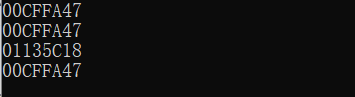2020-02-25 10:31

# C++指针进过函数地址赋值没有传递过去，怎么回事？

`````````void f1(char *p)
{
cout << static_cast<const void *>(p) << endl;
char *a= new char;
p = a;

cout << static_cast<const void *>(p) << endl;
delete a;
}
int main()
{
char *p=NULL;
char d = 'q';
p = &d;
cout << static_cast<const void *>(p)<<endl;
f1(p);
cout << static_cast<const void *>(p) << endl;
return 0;
}
``````• 写回答
• 关注问题
• 收藏
• 邀请回答